Next Article in Journal
An Evidential Prospect Theory Framework in Hesitant Fuzzy Multiple-Criteria Decision-Making
Previous Article in Journal
Complex Asymptotics in λ for the Gegenbauer Functions Cλα(z) and Dλα(z) with z∈(-1,1)
Previous Article in Special Issue
Docking of Polyethylenimines Derivatives on Cube Rhombellane Functionalized Homeomorphs

Symmetry 2019, 11(12), 1466; https://doi.org/10.3390/sym11121466

Article
Riemann-Symmetric-Space-Based Models in Screening for Gene Transfer Polymers
byClaudiu N. Lungu 1,*andIreneusz P. Grudzinski 2
1
Department of Chemistry, Faculty of Chemistry and Chemical Engineering, Babes-Bolyai University, 400028 Cluj, Romania
2
Department of Applied Toxicology, Faculty of Pharmacy, Medical University of Warsaw, 02-097 Warsaw, Poland
*
Author to whom correspondence should be addressed.
Received: 10 October 2019 / Accepted: 27 November 2019 / Published: 1 December 2019

## Abstract

:
Today, gene transfer using polymers as transfer vectors is hardly studied. Some polymers have an excellent gene-carrying ability, but their cytotoxic and biocompatibility properties are not suitable for use. Thus, increased insight into the drug space of such structures is needed in the screening for suitable molecules. This study aimed to introduce a mathematical model of polymers suitable for genes transfer. In this regard, Riemann surfaces were used. The concerned polymers were taken from secondary published experimental data. The results show that symmetric Reimann spaces are suitable for further drug screening. The branch point values of Riemann surfaces are especially increased for the polymers suitable in gene transfer.
Keywords:
polymers; gene transfer; Riemann space; chemical space; manifold; branching point

## 1. Introduction

The lack of gene-delivery vectors  is the main limiting factor in the field of gene personalized therapy . Viral vectors have a low efficiency. Synthetic gene-delivery agents do not possess the required efficacy . In recent years, a variety of capable polymers have been designed, specifically for gene delivery. Understanding the polymer gene-delivery mechanisms will help in the design of polymer-based gene-delivery systems, thus becoming essential tools for human gene therapy. The design criteria for the construction of useful delivery vectors are continuously evolving. Reverse drug design, fragment-based drug design, and virtual screening all failed in identifying a class candidate. Most of these methods explore the same drug space of a template compound. Thus, a mathematical model, expressed as a function, will enlarge the drug ability space. Such a model will go beyond the respective class of compounds. A way of generating such a model is to explore Cartesian coordinates, close contacts, dihedral angle values, and the internal coordinates of a certain molecule. Cartesian coordinates can provide a high starting point for projection in a vast range of mathematical spaces, compared with internal coordinates and close contacts. One can distinguish linear spaces and topological spaces. Linear spaces lack 3D dimensionality due to their algebraic nature. Linear operations performed in a linear space lead to straight lines. Their dimensionality is defined as the maximal number of independent vectors.
Topological spaces that are analytic in nature lead to continuous functions. A topological space is hard to define. An algebraic approach is used in most cases.
Polynomial equations and their geometric properties are used worldwide in algebraic geometry. A major characteristic that makes polynomial equations a valuable tool in topology is their definition from a basic arithmetic operation—addition and multiplication. This operation, when used, retrieves smooth and Riemannian manifolds.
Manifolds are a type of topological space that resembles Euclidian space. The concept of manifolds is a keystone in geometry, while it allows complex structures to be characterized from the perspective of the concept of local topological properties. The cartesian coordinates and dihedral angles of a structure can be viewed as carrying these topological properties.
A smooth manifold is not an actual space. Furthermore, topological manifolds are defined as smooth manifolds that are finite linear spaces. In other words, the surface of an ellipsoid can be represented as a smooth manifold.
Every real structure has its own Euclidean space representation. Riemann manifolds can also be considered to be Euclidean spaces.
Mathematical spaces, usually those in a complex analysis, coexist with drug spaces both in real roots and complex ones . The significant backtracking of these techniques based on molecule topology is the actual force field, i.e., the level of theory used to optimize the respective compound.
Statistical and mathematical models are essential tools in predicting molecular properties. QSAR methodology uses a set of molecules in order to predict one distinct molecular property by computing a set of descriptors based on which a quantitative structure–activity relationship (QSAR) equation is generated. This equation is used to further predict specific properties. The molecular descriptors used in QSAR models are driven from experimental data like logP, molar refractivity, polarizability, and theoretical descriptors, which are generally symbolical representations of a molecule. The theoretical descriptors characterize the constitution of the molecule, structural fragments, the graph invariants, the 3D properties (molecule size, volume), and the properties derived from the grid base molecular force field (GRID), comparative molecular field analysis (CoMFA) and similar methods, respectively. Both types of descriptors, experimental and theoretical, are used in establishing a prediction model. Such models involve a specific chemical space.
Furthermore, the more diversified the molecular set is (i.e., the more the molecules differ in respect to their molecular formula and structure) the more the model will cover a vast chemical space. Thus, by using a diverse set of molecules one will obtain a better prediction model. In other words, the set of molecules used in this study are diverse (i.e., distinct molecular formula, distinct structure) so they can characterize accurately a chemical space, making the model applicable to novel molecules
In this study, an inverse methodology was proposed in order to identify polymers with gene transfer capabilities. Instead of building a QSAR model that will explore a fraction of the chemical space, the chemical space was investigated with the help of the Cartesian coordinates. The equations derived from the Cartesian coordinates were used to compute chemical spaces—Riemannian spaces.
Theoretically, a model built in such a way provides an extensive overview of the drug space, both in real numbers and in imaginary complex number spaces. Such equations (polynomial equations)/models (Riemann spaces) can work as “reverse engineering” and lead to suitable gene-carrying polymers, with good cytotoxicity and biocompatibility profiles.

## 2. Materials and Methods

A set of 29 monomers (Table 1), retrieved from the gene transfer literature , were used to compute a mathematical model. The in silico models in the mol2 format of polymer structures were generated using the Chemoffice 2005 software package . The models were optimized at the 6-31G level of theory . The cartesian coordinates (Figure 1) of polymer structures were used to develop derivate equations of coordinates (Table 2 and Table 3), using Mathematica 5.0 software . The Riemann surfaces for each derived coordinate equation were solved and computed using the same software (Mathematica). The branch point for each equation was computed and represented. A 2D (complex map) and a 3D (Riemann surface) were used to represent the results. In addition, a single hypothesis QSAR model was computed using the Schrodinger 2009 software package . The pharmacophores were computed; having compounds 8 and 21 as templates, they were chosen because of their proven gene transfer properties. Moreover, a combined pharmacophore hypothesis was developed. The branching points were compared against the known gene transfer properties. The methodology is exemplified for compounds 8 and 21.

## 3. Results

Table 2 lists the initial derivate equations generated using the Cartesian coordinates of each molecule.
The equations in Table 2 were transformed using the following formula:
∂H(−a + bH) → z(−a + bz)^(1/(z(−a + bz)))
where a = free term; b = coefficient; z = Lambert W function.
The equations for each polymer are shown in Table 3.
The Riemann surfaces obtained for compounds 8 and 21 that showed promising experimental results are shown in Figure 2.
The branching points computed for each structure are shown in Figure 3.
The QSAR models, developed as a single hypothesis for compounds 8 and 21, and the merge hypothesis are shown in Figure 4.
The Cartesian coordinates of each hypothesis are listed here. Hypothesis 1: D1D2H3H4; D1 (x −4.18, y 2.07, z −0.84); D2 (x −4.48, y −2.07, z 0.84); H3 (x −5.97, y 2.62, z 0.00); H4 (x −2.69 y −2.69, z 0.00).
Hypothesis 2: A1A2A3A4H5H6; A1 (x −3.11, y 3.66, z 0.00); A2 (x 2.06, y −3.25, z 0.00); A3 (x −2.32, y 5.77, z 0.00); A4 (x −2.32, y 5.77, z 0.00); H5 (−1.38, y 1.91, z 0.00); H6 (x 0.35, y −1.50, z 0.00).
Merge hypothesis: A1A2D2D3H5H6; A1 (x −3.11, y 3.66, z 0.00); A2 (x 2.06, y −3.25, z 0.00); D2 (x −4.48, y −2.07, z 0.34); D3 (x −4.18. y 2.07, z −0.84); D4 (x −4.48, y −2.07, z 0.84); H5 (x −5.97, y 2.62, z 0.00); H6 (x −2.69, y −2.62, z 0.00). As observed above, the merge hypothesis has common elements from both hypotheses 1 and 2, but also leaves out D1 and H4 from hypotheses 1 and A3 and A4 from hypothesis 2, respectively.

## 4. Discussion

Symmetric spaces are pseudo-Riemann manifolds . A connected Riemann manifold is symmetric (space) if its curvature tensor is invariant. Broadly, a Riemann manifold is symmetric if each point exists as an isometry. Furthermore, every symmetric space is exhaustive.
Riemann symmetric spaces are considered in physics, mathematics, and chemistry. They have a central role in the homology theory. Examples of Riemann spaces include Euclidean spaces, hyperbolic spaces, and projective spaces. Riemann spaces are classified into the Euclidean type, the Compact type, and the Non-Compact type .
If the complex argument of a function can be mapped from a single point in the domain of multiple points, then the branching point of an analytical function is a point in the complex plane . When z (branching point) = 0, under the power function f(z) = za, where “a” is a complex non-integer (“a” ∈ C, with a ∄ Z). Writing z = eiθ and taking θ in the interval 0 to 2π results in:
f(eoi) = e0 = 1; f(e2πi) = e2πia
so that the values of the function f(z); z = (0;2π) are different [12,13,14,15].
In Riemann surfaces, the aspect of a branch point is defined for a holomorphic function when ƒ: X → Y, from a compact connected Riemann surface X to the compact Riemann surface Y (usually the Riemann sphere). If ƒ is not constant, ƒ will be a covering map onto its image at all but a finite number of points. The points of X, where the function ƒ fails, are the bifurcations points of ƒ, and the branch point is an image of a ramification point under ƒ.
For any point P ∈ X and Q = ƒ(P) ∈ Y, there are the holo-morphic local coordinates z for X near P and w for Y near Q, in terms of which the function ƒ(z) is given by ω = zk, for some integer k. This integer is called the ramification index of P. Usually, the ramification index equals one; if the branching index is not equal to one, then P is, by definition, a ramification point, and Q is a branch point.
If Y is just the Riemann sphere, and Q is in the finite part of Y, then there is no demand to select particular coordinates. The ramification index (Equation (3)) can be determined explicitly from Cauchy’s integral formula. Let γ be a simple rectifiable loop in X around P. The ramification index of ƒ at P is (P-any point in the space with local holomorphic coordinates)
$e P = 1 2 π i ∫ y f ′ ( z ) f ( z ) − f ( P ) d z$
This integral is the number of times that ƒ(y) winds over the point Q. As above, P is a ramification point, and Q is a branch point if eP > 1.
A Riemann surface is a surface-like composition that encloses the complex plane with infinitely many “sheets.” These sheets can have very intricate structures and interconnections. Riemann surfaces are one way of representing multiple-valued functions; another way is represented by the branch cuts. The plot in Figure 1 shows the Riemann surfaces as the solutions of the equation:
[w(z)]^d + w(z) + z^(d−1) = 0
with d = 2, 3, 4, and 5, where w(z) is the Lambert W-function.
The Riemann surface S of the function field K is the set of non-trivial discrete evaluations on K. Here, the set S corresponds to the ideals of the ring A of integers of K over z. Riemann surfaces provide a geometric visualization of the function elements and their analytical continuations.
Schwarz proved, at the end of the nineteenth century, that the automorphism group of a unified Riemann surface of genus g ≥ 2 is finite; then, Hurwitz showed that the group order is at most 84 (g − 1), where “g” is the genus.
In light of the computational results, polymers #4, 8, 11, 16 and 21, are the best candidates for feasible gene transfer. Experimentally, only polymers 8 and 21 showed good and acceptable results. A threshold regarding the branching point and its correlation with bioactivity was observed (Figure 2). A branching point of ~1 has an excellent correlation with gene transfer capability, cytotoxicity, and biocompatibility.
The QSAR single hypothesis models for compounds 8 and 21 revealed the importance of topology in performing bioactivity. The merge hypothesis presents a shared future for both compounds 8 and 21. Hydrogen atom accepting A-groups and donor D-groups are critical in the chemical space of compounds . Furthermore, the pharmacophores demonstrated a relatively diverse set of functional groups for each hypothesis, findings that suggest a lack of specificity in describing a common pharmacophore.
Lastly, using the characteristics of symmetric mathematical space , one can characterize distinct molecular properties, as shown in Table 4, where the Riemman spaces for compounds 8 and 21 are represented with their complex aspects (see Supplementary Material S2) [18,19,20].

## 5. Conclusions

A suitable mathematical model, based on Riemann surfaces can be built in order to screen and characterize polymers with gene transfer properties. The branching point of Riemann surfaces can aid in assessing such surfaces, their connection with the drug space and their connective properties.

## Supplementary Materials

The following are available online at https://www.mdpi.com/2073-8994/11/12/1466/s1. S2—Riemann surfaces.

## Author Contributions

C.N.L. established the conceptual framework, produced the results and discussion, and assembled the paper. I.P.G. performed the literature screening of concerned compounds and methods and contributed the references and the result discussion.

## Funding

This work was supported by the GEMNS project granted in the European Union’s Seventh Framework Program under ERA-NET EuroNanoMed II (European Innovative Research and Technological Development Projects in Nanomedicine).

## Acknowledgments

We are especially indebted to all reviewers for graciously going through these drafts and making numerous substantive corrections and suggestions about how to improve the quality of the paper. We are especially indebted to Sohan Jheeta (of The Network of Researchers on the Chemical Evolution of Life, NoR CEL, www.nor-cel.com) for graciously going through several drafts and making numerous substantive suggestions about how to improve the quality of the paper.

## Conflicts of Interest

The authors declare no conflict of interest.

## References

1. Mary, B.; Maurya, S.; Kumar, M.; Bammidi, S.; Kumar, V.; Jayandharan, G.R. Molecular engineering of Adeno-associated virus capsid improves its therapeutic gene transfer in murine models of hemophilia and retinal degeneration. Mol. Pharm. 2019, 16, 4738–4750. [Google Scholar] [CrossRef] [PubMed]
2. Aartsma-Rus, A.; van Putten, M. The use of genetically humanized animal models for personalized medicine approaches. Dis. Model. Mech. 2019, 13, 041673. [Google Scholar] [CrossRef] [PubMed]
3. Contin, M.; Garcia, C.; Dobrecky, C.; Lucangioli, S.; D’Accorso, N. Advances in drug delivery, gene delivery and therapeutic agents based on dendritic materials. Future Med. Chem. 2019, 11, 1791–1810. [Google Scholar] [CrossRef] [PubMed]
4. Richard, B.; Ho-Fung, C.; Jóhannes, R. Characteristics of known drug space. Natural products, their derivatives and synthetic drugs. Eur. J. Med. Chem. 2010, 45, 5646–5652. [Google Scholar]
5. Anderson, D.G.; Peng, W.; Akinc, A.; Naushad, H.; Kohn, A.; Pandera, R.; Langer, R.; Sawicli, A.J. A polymer library approach to suicide gene therapy for cancer. Proc. Natl. Acad. Sci. USA 2004, 9, 16028–16033. [Google Scholar] [CrossRef] [PubMed]
6. Beata, S.; Mircea, V.D.; Mihai, V.P.; Ireneusz, P.G. Docking linear ligands to glucose oxidase. Int. J. Mol. Sci. 2016, 17, 1796. [Google Scholar]
7. Nguyen, L.H.; Nguyen, T.H.; Truong, T.N. Quantum Mechanical-Based Quantitative Structure-Property Relationships for Electronic Properties of Two Large Classes of Organic Semiconductor Materials: Polycyclic Aromatic Hydrocarbons and Thienoacenes. ACS Omega 2019, 4, 7516–7523. [Google Scholar] [CrossRef] [PubMed]
8. Mathematica; Version 8.0; Wolfram Research, Inc.: Champaign, IL, USA, 2010.
9. Schrödinger Release Prime; Schrödinger LLC: New York, NY, USA, 2009.
10. Akhiezer, D.N.; Vinberg, E.B. Weakly symmetric spaces and spherical varieties. Transf. Groups 1999, 4, 3–24. [Google Scholar] [CrossRef]
11. Wolf Joseph, A. Spaces of Constant Curvature, 5th ed.; McGraw–Hill: New York, NY, USA, 1999. [Google Scholar]
12. Conway John, B. Functions of One Complex Variable, 2nd ed.; Springer: Berlin/Heidelberg, Germany, 1978. [Google Scholar]
13. Arfken, G. Mathematical Methods for Physicists, 3rd ed.; Academic Press: Orlando, FL, USA, 1985; pp. 397–399. [Google Scholar]
14. Morse, P.M.; Feshbach, H. Methods of Theoretical Physics, Part I; McGraw-Hill: New York, NY, USA, 1953; pp. 391–392, 399–401. [Google Scholar]
15. Trott, M. The Mathematica Guidebook for Programming; Springer: Berlin/Heidelberg, Germany, 2004; pp. 188–191. [Google Scholar]
16. Weisstein, E.W. “Branch Point” from MathWorld—A WolframWeb Resource. Available online: http://mathworld.wolfram.com/BranchPoint.html (accessed on 5 December 2018).
17. Majumdar, S.; Basak, S.C.; Lungu, C.N.; Diudea, M.V.; Grunwald, G.D. Mathematical structural descriptors and mutagenicity assessment: A study with congeneric and diverse datasets. SAR QSAR Environ. Res. 2018, 29, 579–590. [Google Scholar] [CrossRef] [PubMed]
18. Petitjean, M. A definition of symmetry. Symmetry Cult. Sci. 2007, 18, 99–119. [Google Scholar]
19. Lungu, C.N. C-C chemokine receptor type 3 inhibitors: Bioactivity prediction using local vertex invariants based on thermal conductivity layer matrix. Stud. Univ. Babes-Bolyai Chem. 2018, 63, 177–188. [Google Scholar] [CrossRef]
20. Lungu, C.N.; Diudea, M.V.; Putz, M.V. Ligand shaping in induced fit docking of MraY inhibitors. Polynomial discriminant and Laplacian operator as biological activity descriptors. Int. J. Mol. Sci. 2018, 18, 1377. [Google Scholar] [CrossRef] [PubMed]
Figure 1. Cartesian coordinates of compounds 8 and 21.
Figure 1. Cartesian coordinates of compounds 8 and 21.
Figure 2. Riemann surfaces for compounds 8 and 21 respectively with their respective branching point values.
Figure 2. Riemann surfaces for compounds 8 and 21 respectively with their respective branching point values.
Figure 3. Branching points for the concerned monomers; points of structures with promising gene transfer profile, in red (see Supplementary Material S1).
Figure 3. Branching points for the concerned monomers; points of structures with promising gene transfer profile, in red (see Supplementary Material S1).
Figure 4. (a) Pharmacophore hypothesis based on molecule #8; (b) pharmacophore hypothesis based on molecule #21; (c) merge pharmacophore hypothesis (a + b): Lyophilic (H-atom group, in green); hydrogen donor group (D, in blue); hydrogen acceptor group (A, in red).
Figure 4. (a) Pharmacophore hypothesis based on molecule #8; (b) pharmacophore hypothesis based on molecule #21; (c) merge pharmacophore hypothesis (a + b): Lyophilic (H-atom group, in green); hydrogen donor group (D, in blue); hydrogen acceptor group (A, in red).
Table 1. Monomers with proven gene transfer capabilities.
Table 1. Monomers with proven gene transfer capabilities.
ItemMonomers with Proven Gene Transfer Capabilities
12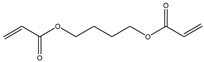345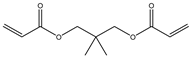67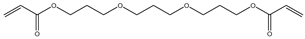89101111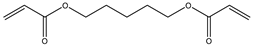121314151617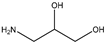1819202122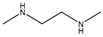2324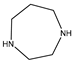25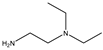26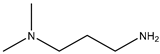27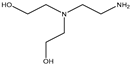2829Table 2. Derived equations based on the Cartesian coordinates, where H is the independent variable.
Table 2. Derived equations based on the Cartesian coordinates, where H is the independent variable.
Monomer Equations
#Coordinate Based Equations
1H(−13.4 + 19H)
2H(−2.2 + 18.5H)
3H(−13.0 + 28H)
4H(−13.6 + 14H)
5H(−2.4 + 15H)
6H(−2.6 + 15H)
7H(−4.9 + 17H)
8H(−15.5 + 20H)
9H(−4.5 + 23H)
10H(−4.3 + 18H)
11H(−19.1 + 24H)
12H(−3.4 + 19H)
13H(−3.7 + 24H)
14H(−2.4 + 21H)
15H(−4.6 + 26H)
16H(−4.0 + 2.8H)
17H(6.8H)
18H(−1.8 + 19H)
19H(−1.9 + 795.2H)
20H(−13.4 + 28H)
21H(−28.7 + 28H)
22H(−16.7 + 59H)
23H(83.1H)
24H(−10.8 + 31H)
25H(25H)
26H(31H)
27H(−7.3 + 323.5H)
28H(398.6H)
29H(−5.8 + 49H)
Table 3. Distinct equations for each compound based on the Cartesian coordinates.
Table 3. Distinct equations for each compound based on the Cartesian coordinates.
#Equations for Computing Riemann Surfaces
1z (−13.4 + 19 z)^(1/(z (−13.4 + 19 z)))
2z (−2.2 + 18.5z)^(1/(z (−2.2 + 18.5z)))
3z (−13.0 + 28z)^(1/(z (−13.0 + 28z)))
4z (−13.6 + 14 z)^(1/(z (−13.6 + 14z)))
5z (−2.4 + 15 z)^(1/(z (−2.4 + 15 z)))
6z (−2.6 + 15z)^(1/(z (−2.6 + 15z)))
7z (−4.9 + 17z)^(1/(z (−4.9 + 17z)))
8z (−15.5 + 20z)^(1/(z (−15.5 + 20z)))
9z (−4.5 + 23z)^(1/(z (−4.5 + 23 z)))
10z (−4.3 + 18z)^(1/(z (−4.3 + 18z)))
11z (−19.1 + 24z)^(1/(z (−19.1 + 24z)))
12z (−3.4 + 19 z)^(1/(z (−3.4 + 19z)))
13z (−3.7 + 24 z)^(1/(z (−3.7 + 24z)))
14z (−2.4 + 21z)^(1/(−2.4 + 21 z)))
15z (−4.6 + 26z)^(1/(z (−4.6 + 26 z)))
16z (−4.0 + 2.8 z)^(1/(z (−4.0 + 28 z)))
17z (6.8 z)^(1/(z (6.8 z)))
18z (−1.8 + 19z)^(1/(z (−1.8 + 19 z)))
19z (−1.9 + 795.2 z)^(1/(z (−1.9 + 795.2 z)))
20z (−13.4 + 28 z)^(1/(z (−13.4 + 28 z)))
21z (−28.7 + 28 z)^(1/(z (−28.7 + 28 z)))
22z (−16.7 + 59 z)^(1/(z (−16.7 + 59 z)))
23z ( 83.1 z)^(1/(z (83.1 z)))
24z (−10.8 + 31 z)^(1/(z (−10.8 + 31 z)))
25z (25 z)^(1/(z ( 25 z)))
26z (31 z)^(1/(z ( 31z)))
27z (−7.3 + 323.5 z)^(1/(z (−7.3 + 323.5 z)))
28z ( 398.6 z)^(1/(z (398.6 z)))
29z (−5.8 + 49 z)^(1/(z (−5.8 + 49 z)))
Table 4. Riemann surfaces: 1—function plots, 2—complex space plots, 3—real part 3D plot, 4—imaginary part 3D plot, 5—branching number, and 6—polymers (#).
Table 4. Riemann surfaces: 1—function plots, 2—complex space plots, 3—real part 3D plot, 4—imaginary part 3D plot, 5—branching number, and 6—polymers (#).
Riemann Surfaces
1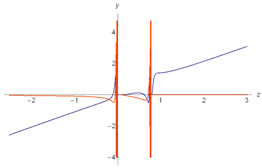2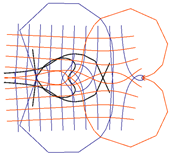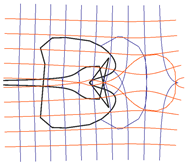3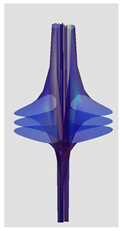450.77541.027
6# 8# 21